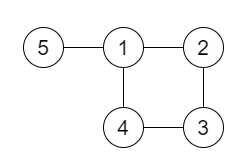# Redundant Connection in C++

Suppose we have one unrooted tree; this is one undirected graph with no cycles. The given input is a graph that started as a tree with N nodes (values of the nodes are distinct values ranging from 1 through N), with one additional edge added. The added edge has two different vertices chosen from 1 to N, and was not an edge that already existed.

The final graph is given as a 2D-array of edges. Each element of edges is a pair [u, v] where u < v, that represents an undirected edge connecting nodes u and v.

We have to find an edge that can be removed so that the resulting graph is a tree of N nodes. There may be multiple answers, we have to find the answer that occurs last in the given 2Darray. The answer edge [u, v] should be in the same format, with u < v.

So, if the input is like [[1,2], [2,3], [3,4], [1,4], [1,5]],then the output will be [1,4]

To solve this, we will follow these steps −

• N := 1000

• Define an array parent of size: N+5.

• Define an array rank of size: N+5.

• Define a function getParent(), this will take n,

• if parent[n] is same as -1, then −

• return n

• return parent[n] = getParent(parent[n])

• Define a function unionn(), this will take a, b,

• pa := getParent(a), pb := getParent(b)

• if pa is same as pb, then −

• return false

• if rank[pa] > rank[pb], then −

• rank[pa] := rank[pa] + rank[pb]

• parent[pb] := pa

• Otherwise

• rank[pb] := rank[pb] + rank[pa]

• parent[pa] := pb

• return true

• From the main method, do the following −

• n := size of edges list

• for initialize i := 0, when i < n, update (increase i by 1), do −

• parent[edges[i, 0]] := -1, parent[edges[i, 1]] := - 1

• rank[edges[i, 0]] := -1, rank[edges[i, 1]] := 1

• Define an array ans

• for initialize i := 0, when i < n, update (increase i by 1), do −

• u := edges[i, 0]

• v := edges[i, 1]

• if unionn(u, v) is zero, then −

• ans := edges[i]

• return ans

## Example

Let us see the following implementation to get better understanding −

Live Demo

#include <bits/stdc++.h>
using namespace std;
void print_vector(vector v){
cout << "[";
for(int i = 0; i<v.size(); i++){
cout << v[i] << ", ";
}
cout << "]"<<endl;
}
const int N = 1000;
class Solution {
public:
int parent[N + 5];
int rank[N + 5];
int getParent(int n){
if (parent[n] == -1)
return n;
return parent[n] = getParent(parent[n]);
}
bool unionn(int a, int b){
int pa = getParent(a);
int pb = getParent(b);
if (pa == pb)
return false;
if (rank[pa] > rank[pb]) {
rank[pa] += rank[pb];
parent[pb] = pa;
}
else {
rank[pb] += rank[pa];
parent[pa] = pb;
}
return true;
}
vector<int> findRedundantConnection(vector<vector<int>>& edges) {
int n = edges.size();
for (int i = 0; i < n; i++) {
parent[edges[i]] = parent[edges[i]] = -1;
rank[edges[i]] = rank[edges[i]] = 1;
}
vector<int> ans;
for (int i = 0; i < n; i++) {
int u = edges[i];
int v = edges[i];
if (!unionn(u, v)) {
ans = edges[i];
}
}
return ans;
}
};
main(){
Solution ob;
vector<vector<int>> v = {{1,2}, {2,3}, {3,4}, {1,4}, {1,5}};
print_vector(ob.findRedundantConnection(v));
}

## Input

{{1,2}, {2,3}, {3,4}, {1,4}, {1,5}}

## Output

[1, 4, ]